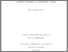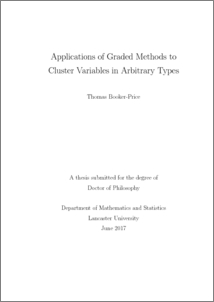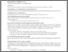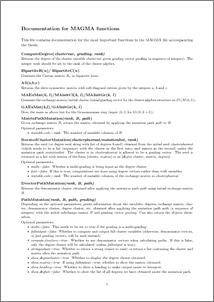# Applications of graded methods to cluster variables in arbitrary types

Booker-Price, Thomas (2017) Applications of graded methods to cluster variables in arbitrary types. PhD thesis, UNSPECIFIED.Preview
PDF (2017Booker-PricePhD)
2017Booker_PricePhD.pdf - Published VersionPreview
PDF (2017Booker-Price_Code_Documentation)
2017Booker_Price_Code_Documentation.pdf - Published Version

Other (2017Booker-Price_code)
2017Booker_Price_code.magma - Published Version

## Abstract

This thesis is concerned with studying the properties of gradings on several examples of cluster algebras, primarily of infinite type. We start by considering two classes of finite type cluster algebras: those of type Bn and Cn. We give the number of cluster variables of each occurring degree and verify that the grading is balanced. These results complete a classification in  for coefficient-free finite type cluster algebras. We then consider gradings on cluster algebras generated by 3×3 skew-symmetric matrices. We show that the mutation-cyclic matrices give rise to gradings in which all occurring degrees are positive and have only finitely many associated cluster variables (excepting one particular case). For the mutation-acyclic matrices, we prove that all occurring degrees have infinitely many variables and give a direct proof that the gradings are balanced. We provide a condition for a graded cluster algebra generated by a quiver to have infinitely many degrees, based on the presence of a subquiver in its mutation class. We use this to study the gradings on cluster algebras that are (quantum) coordinate rings of matrices and Grassmannians and show that they contain cluster variables of all degrees in N. Next we consider the finite list (given in ) of mutation-finite quivers that do not correspond to triangulations of marked surfaces. We show that A(X7) has a grading in which there are only two degrees, with infinitely many cluster variables in both. We also show that the gradings arising from Ee6, Ee7 and Ee8 have infinitely many variables in certain degrees. Finally, we study gradings arising from triangulations of marked bordered 2- dimensional surfaces (see ). We adapt a definition from  to define the space of valuation functions on such a surface and prove combinatorially that this space is isomorphic to the space of gradings on the associated cluster algebra. We illustrate this theory by applying it to a family of examples, namely, the annulus with n + m marked points. We show that the standard grading is of mixed type, with finitely many variables in some degrees and infinitely many in the others. We also give an alternative grading in which all degrees have infinitely many cluster variables.

Item Type:
Thesis (PhD)
Subjects:
Departments:
ID Code:
88866
Deposited By:
Deposited On:
21 Nov 2017 20:34
Refereed?:
No
Published?:
Published